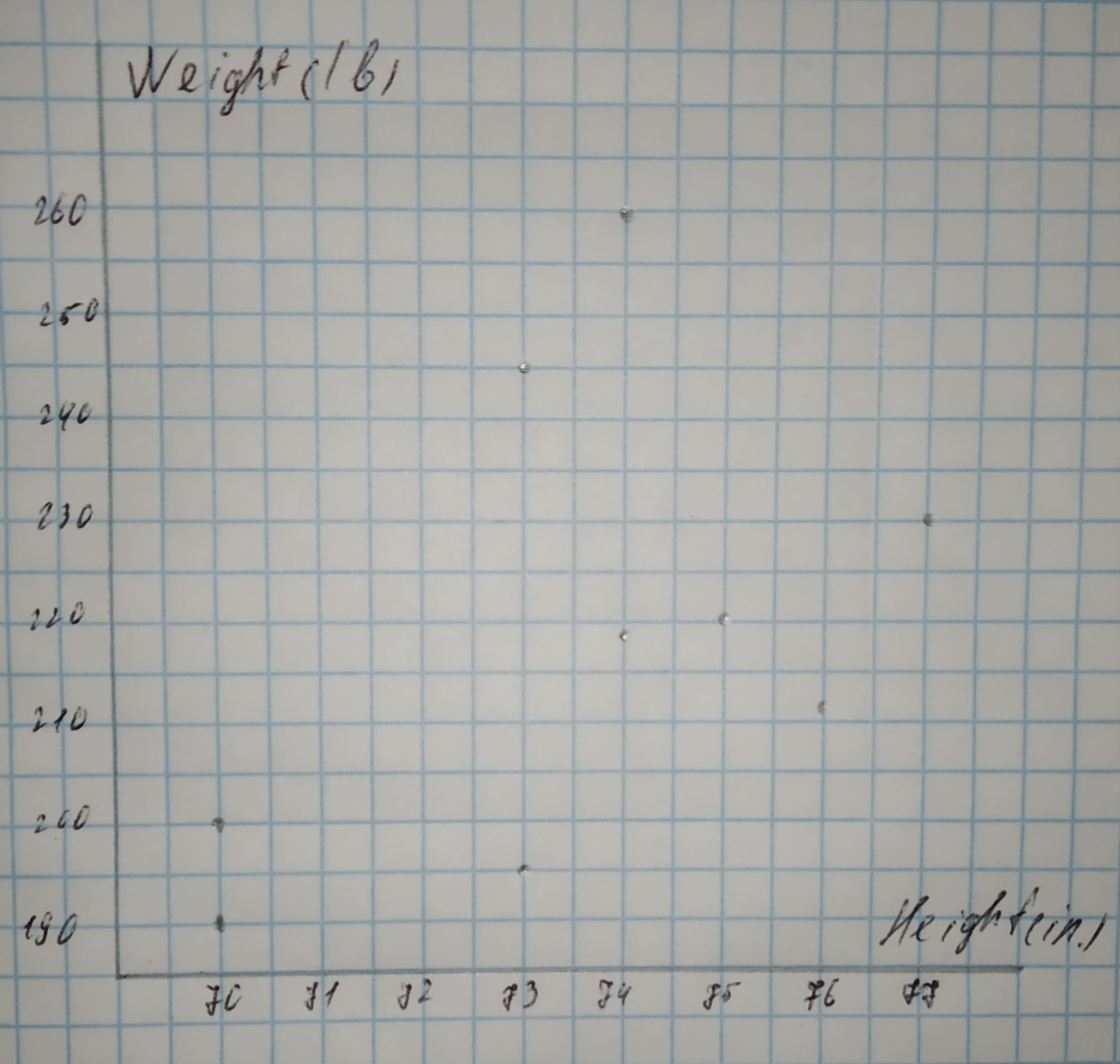Anonym

2021-02-25

a. Make a scatterplot for the data in the table below.
Height and Weight of Football Players
$\begin{array}{|cccccccccc|}\hline \text{Height (in.):}& 77& 75& 76& 70& 70& 73& 74& 74& 73\\ \text{Weight (lb):}& 230& 220& 212& 190& 201& 245& 218& 260& 196\\ \hline\end{array}$

b. Which display - the table or the scatter plot - do you think is a more appropriate display of the data? Explain your reasoning.Fatema Sutton

Step 1
(a) Scatterplot
Height is on the horizontal axis and Weight is on the vertical axis.
The heights range from 70 to 77, thus an appropriate scale for the horizontal axis is from 69 to 78
The weights range from 190 to 260, thus an appropriate scale for the vertical axis is from 180 to 270.Step 2
(b) The scatterplot is a more appropriate display of the data, because the relationship between height and weight is more obvious from the scatterplot compared to the table.
For example, we note that the weight tends to increase as the height increases, because the pattern in the scatterplot slopes upwards. However, this fact would have been much harder to derive from the table.
Result:
(a) Height is on the horizontal axis and Weight is on the vertical axis.
(b) Scatter plot.

Do you have a similar question?